# Introduction to Reluctant Generalized Additive Modeling (RGAM)

## Introduction

relgam is a package that fits reluctant generalized additive models (RGAM), a new method for fitting sparse generalized additive models (GAM). RGAM is computationally scalable and works with continuous, binary, count and survival data.

We introduce some notation that we will use throughout this vignette. Let there be $$n$$ observations, each with feature vector $$x_i \in \mathbb{R}^p$$ and response $$y_i$$. Let $$\mathbf{X} \in \mathbb{R}^{n \times p}$$ denote the overall feature matrix, and let $$y \in \mathbb{R}^n$$ denote the vector of responses. Let $$X_j \in \mathbb{R}^n$$ denote the $$j$$th column of $$\mathbf{X}$$.

Assume that the columns of $$\mathbf{X}$$, i.e. $$X_1, \dots, X_p$$, are standardized to have sample standard deviation $$1$$. Assume that we have specified a scaling hyperparmeter $$\gamma \in [0,1]$$, a degrees of freedom hyperparameter $$d$$, and a path of tuning parameters $$\lambda_1 > \dots > \lambda_m \geq 0$$. RGAM’s model-fitting algorithm, implemented in the function rgam(), consists of 3 steps:

1. Fit the lasso of $$y$$ on $$\mathbf{X}$$ to get coefficients $$\hat{\beta}$$. Compute the residuals $$r = y - \mathbf{X} \hat{\beta}$$, using the $$\lambda$$ hyperparameter selected by cross-validation.

2. For each $$j \in \{ 1, \dots, p \}$$, fit a $$d$$-degree smoothing spline of $$r$$ on $$X_j$$ which we denote by $$\hat{f}_j$$. Rescale $$\hat{f}_j$$ so that $$\overline{\text{sd}}(\hat{f}_j) = \gamma$$. Let $$\mathbf{F} \in \mathbb{R}^{n \times p}$$ denote the matrix whose columns are the $$\hat{f}_j(X_j)$$’s.

3. Fit the lasso of $$y$$ on $$\mathbf{X}$$ and $$\mathbf{F}$$ for the path of tuning parameters $$\lambda_1 > \dots > \lambda_m$$.

Steps 1 and 3 are implemented using glmnet::glmnet() while Step 2 is implemented using stats::smooth.spline(). (Note that the path of tuning parameters $$\lambda_1 > \dots > \lambda_m$$ are only used in Step 3; for Step 1 we use glmnet::glmnet()’s default lambda path.) We will refer to these 3 steps by their numbers (e.g. “Step 1”) throughout the rest of the vignette.

The rgam() function fits this model and returns an object with class “rgam”. The relgam package includes methods for prediction and plotting for “rgam” objects, as well as a function which performs $$k$$-fold cross-validation for rgam().

For more details, please see our paper on arXiv.

## Installation

The simplest way to obtain relgam is to install it directly from CRAN. Type the following command in R console:

install.packages("relgam")

This command downloads the R package and installs it to the default directories. Users may change add a repos option to the function call to specify which repository to download from, depending on their locations and preferences.

Alternatively, users can download the package source at CRAN and type Unix commands to install it to the desired location.

## The rgam() function

The purpose of this section is to give users a general sense of the rgam() function, which is the workhorse of this package. First, we load the relgam package:

library(relgam)

Let’s generate some data:

set.seed(1)
n <- 100; p <- 12
x = matrix(rnorm((n) * p), ncol = p)
f4 = 2 * x[,4]^2 + 4 * x[,4] - 2
f5 = -2 * x[, 5]^2 + 2
f6 = 0.5 * x[, 6]^3
mu = rowSums(x[, 1:3]) + f4 + f5 + f6
y = mu + sqrt(var(mu) / 4) * rnorm(n)

We fit the model using the most basic call to rgam():

fit <- rgam(x, y, verbose = FALSE)
#> init_nz not specified: setting to default (all features)
#> using default value of gamma for RGAM: 0.6

(If verbose = TRUE (default), model-fitting is tracked with a progress bar in the console. For the purposes of this vignette, we will be setting verbose = FALSE.)

rgam() has an init_nz option which (partially) determines which columns in the $$\mathbf{X}$$ matrix will have non-linear features computed in Step 2 of the RGAM algorithm. $$X_j$$ will have a non-linear feature computed for it if (i) it was one of the features selected by cross-validation in Step 1, or (ii) it is one of the indices specified in init_nz. By default, init_nz = 1:ncol(x), i.e. non-linear features are computed for all the original features. Another sensible default is init_nz = c(), i.e. non-linear features computed for just those selected in Step 1. (This version of the RGAM algorithm is denoted by RGAM_SEL in our paper.)

Below, we fit the model with a different init_nz value:

fit <- rgam(x, y, init_nz = c(), verbose = FALSE)
#> using default value of gamma for RGAM_SEL: 0.8

You might have noticed that in both cases above, we did not specify a value for the gamma hyperparameter: rgam() chose one for us and informed us of the choice. The default value for gamma is 0.6 if init_nz = c(), and is 0.8 in all other cases.

The degrees of freedom hyperparameter for Step 2 of the RGAM algorithm can be set through the df option, the default value is 4. Here is an example of fitting the RGAM model with different hyperparameters:

fit <- rgam(x, y, gamma = 0.6, df = 8, verbose = FALSE)
#> init_nz not specified: setting to default (all features)

The function rgam() fits a RGAM for a path of lambda values and returns a rgam object. Typical usage is to have rgam() specify the lambda sequence on its own. The returned rgam object contains some useful information on the fitted model. For a given value of the $$\lambda$$ hyperparameter, RGAM gives the predictions of the form

$$\hat{y} = \sum_{j=1}^p \hat{\alpha}_j X_j + \sum_{j = 1}^p \hat{\beta}_j \hat{f}_j(X_j),$$

where $$\hat{f}_j(X_j)$$ are the non-linear features constructed in Step 2, while the $$\hat{\alpha}_j$$ and $$\hat{\beta}_j$$ are the fitted coefficients from Step 3. The returned RGAM object has nzero_feat, nzero_lin and nzero_nonlin keys which tell us how many features, linear components and non-linear components were included in the model respectively. (In mathematical notation, nzero_lin and nzero_nonlin count the number of non-zero $$\hat{\alpha}_j$$ and $$\hat{\beta}_j$$ respectively, while nzero_feat counts the number of $$j$$ such that at least one of $$\hat{\alpha}_j$$ and $$\hat{\beta}_j$$ is non-zero).

# no. of features/linear components/non-linear components for 20th lambda value
fit$nzero_feat #>  7 fit$nzero_lin
#> s19
#>   5
fit$nzero_nonlin #> s19 #> 5 While the nzero_feat, nzero_lin and nzero_nonlin keys tell us the number of features, linear components and non-linear components included for each lambda value, the feat, linfeat and nonlinfeat tell us the indices of these features or components. # features included in the model for 20th lambda value fit$feat[]
#>   2  3  4  5  6 10 11
# features which have a linear component in the model for 20th lambda value
fit$linfeat[] #>  2 3 4 5 6 # features which have a non-linear component in the model for 20th lambda value fit$nonlinfeat[]
#>   4  5  6 10 11

In general, we have fit$nzero_feat[[i]] == length(fit$feat[[i]]), fit$nzero_lin[[i]] == length(fit$linfeat[[i]]) and fit$nzero_nonlin[[i]] == length(fit$nonlinfeat[[i]]).

## Predictions

Predictions from this model can be obtained by using the predict method of the rgam() function output: each column gives the predictions for a value of lambda.

# get predictions for 20th model for first 5 observations
predict(fit, x[1:5, ])[, 20]
#>   1.408575 -3.099603  7.362201 -1.609205  6.729858

The getf() function is a convenience function that gives the component of the prediction due to one input variable. That is, if RGAM gives predictions

$$\hat{y} = \sum_{j=1}^p \hat{\alpha}_j X_j + \sum_{j = 1}^p \hat{\beta}_j \hat{f}_j(X_j),$$

then calling getf() on $$X_j$$ returns $$\hat{\alpha}_j X_j + \hat{\beta}_j \hat{f}_j(X_j)$$. For example, the code below gives the component of the response due to variable 5 at the 20th lambda value:

f5 <- getf(fit, x, j = 5, index = 20)
as.vector(f5)
#>     -0.13038873  -2.00876503   1.96362206   1.93205913   1.94826187
#>      1.92201185   1.91597986   1.86679777  -0.64901723   1.87631654
#>     1.93028602   0.18113054  -0.18964651   1.76235317   1.98516408
#>     0.33605522  -0.07207409   1.83242679  -0.89754110  -1.29738244
#>    -0.63653216   1.85948754   1.60090148  -2.98736659   0.27251312
#>    -1.02410778  -2.21467504   1.74899872   1.93616023   1.89608549
#>    -4.76717869   0.37014025  -1.53780332   1.52676873   0.24381746
#>     1.78444567   0.01999517   1.52816257   1.93043905   0.18697503
#>     1.95858387  -1.51026593   1.51357019   0.04242528   0.99736549
#>   -12.45969898  -0.51655212   1.95300610   0.91815634  -6.95724959
#>    -0.02530384   1.96278776   1.79530566  -1.69059815  -1.04489654
#>     1.35644755   0.35729116  -5.20859065   1.17565474   0.31850577
#>     1.96844763  -3.44438244  -0.34551050   0.24086324   1.46691069
#>     0.81357489   1.86548080   0.20517806  -0.72324681  -0.14052225
#>     1.68488945  -2.09699455   1.67367201   0.46966183  -0.49447192
#>    -0.24748950   0.61925974   0.92639442  -0.27793468   1.53241240
#>     1.90969419   0.61303031   1.98600575   1.91294888  -4.52315844
#>    -5.05399415   1.75016795   1.03578280  -4.36019460   0.34930944
#>     0.85309077  -4.14328595   1.52350923   1.22980304 -10.49335410
#>     0.77723033   0.74645435   0.78568544   0.65539011   1.55355891

We can use this to make a plot showing the effect of variable 5 on the response:

plot(x[, 5], f5, xlab = "x5", ylab = "f(x5)")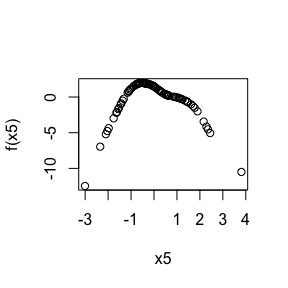(The “Plots and summaries” section shows how to get such a plot more easily.)

## Plots and summaries

Let’s fit the basic rgam model again:

fit <- rgam(x, y, verbose = FALSE)
#> init_nz not specified: setting to default (all features)
#> using default value of gamma for RGAM: 0.6

fit is a class “rgam” object which comes with a plot method. The plot method shows us the relationship our predicted response has with each input feature, i.e. it plots $$\hat{\alpha}_j X_j + \hat{\beta}_j \hat{f}_j(X_j)$$ vs. $$X_j$$ for each $$j$$. Besides passing fit to the plot() call, the user must also pass an input matrix x: this is used to determine the coordinate limits for the plot. It is recommended that the user simply pass in the same input matrix that the RGAM model was fit on.

By default, plot() gives the fitted functions for the last value of the lambda key in fit, and gives just the plots for the first 4 features:

par(mfrow = c(1, 4))
par(mar = c(4, 2, 2, 2))
plot(fit, x)
#> Warning in plot.rgam(fit, x): Plotting first 4 variables by default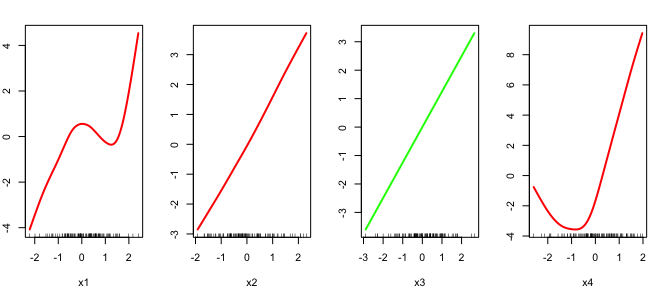The user can specify the index of the lambda value and which feature plots to show using the index and which options respectively:

# show fitted functions for x2, x5 and x8 at the model for the 15th lambda value
par(mfrow = c(1, 3))
plot(fit, x, index = 15, which = c(2, 5, 8))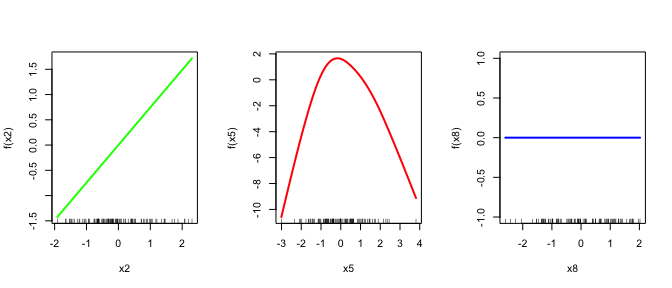Linear functions are colored green, non-linear functions are colored red, while zero functions are colored blue.

Class “rgam” objects also have a summary method which allows the user to see the coefficient profiles of the linear and non-linear features. On each plot (one for linear features and one for non-linear features), the x-axis is the $$\lambda$$ value going from large to small and the y-axis is the coefficient of the feature.

summary(fit)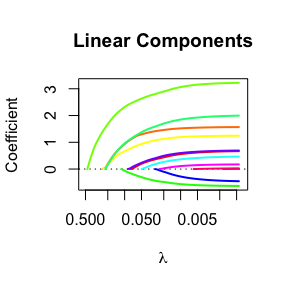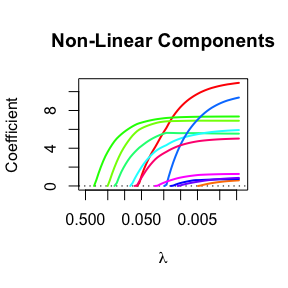By default the coefficient profiles are plotted for all the variables. We can plot for just a subset of the features by specifying the which option. We can also include annotations to show which profile belongs to which feature by specifying label = TRUE.

# coefficient profiles for just features 4 to 6
summary(fit, which = 1:6, label = TRUE)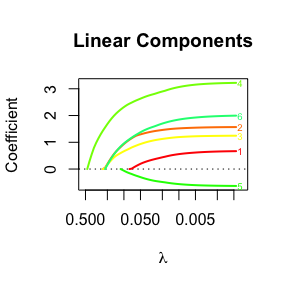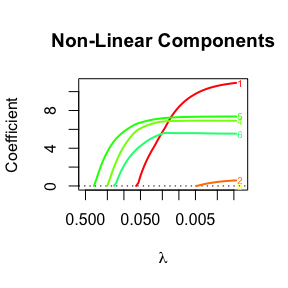## Cross-validation (CV)

We can perform $$k$$-fold cross-validation (CV) for RGAM with cv.rgam(). It does 10-fold cross-validation by default:

cvfit <- cv.rgam(x, y, init_nz = 1:ncol(x), gamma = 0.6, verbose = FALSE)

We can change the number of folds using the nfolds option:

cvfit <- cv.rgam(x, y, init_nz = 1:ncol(x), gamma = 0.6, nfolds = 5, verbose = FALSE)

If we want to specify which observation belongs to which fold, we can do that by specifying the foldid option, which is a vector of length $$n$$, with the $$i$$th element being the fold number for observation $$i$$.

set.seed(3)
foldid <- sample(rep(seq(10), length = n))
cvfit <- cv.rgam(x, y, init_nz = 1:ncol(x), gamma = 0.6, foldid = foldid, verbose = FALSE)

A cv.rgam() call returns a cv.rgam object. We can plot this object to get the CV curve with error bars (one standard error in each direction). The left vertical dotted line represents lambda.min, the lambda value which attains minimum CV error, while the right vertical dotted line represents lambda.1se, the largest lambda value with CV error within one standard error of the minimum CV error.

plot(cvfit)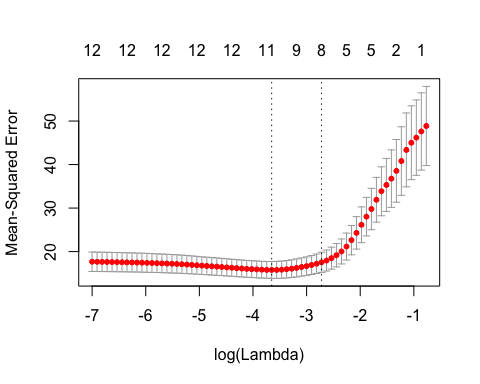The numbers at the top represent the number of features in our original input matrix that are included in the model (i.e. the number of $$j$$ such that at least one of $$\hat{\alpha}_j$$ and $$\hat{\beta}_j$$ is non-zero).

The two special lambda values can be extracted directly from the cv.rgam object as well:

cvfit$lambda.min #>  0.02595555 cvfit$lambda.1se
#>  0.06580678

Predictions can be made from the fitted cv.rgam object. By default, predictions are given for lambda being equal to lambda.1se. To get predictions are lambda.min, set s = "lambda.min".

predict(cvfit, x[1:5, ])   # s = lambda.1se
#>              1
#> [1,]  1.863440
#> [2,] -4.477733
#> [3,]  8.664422
#> [4,] -0.874814
#> [5,]  6.672235
predict(cvfit, x[1:5, ], s = "lambda.min")
#>              1
#> [1,]  1.567028
#> [2,] -4.516911
#> [3,]  9.324387
#> [4,] -1.443938
#> [5,]  8.087449

## RGAM for other families

In the examples above, y was a quantitative variable (i.e. takes values along the real number line). As such, using the default family = "gaussian" for rgam() was appropriate. The RGAM algorithm, however, is very flexible and can be used when y is not a quantitative variable.

rgam() is currently implemented for three other family values: "binomial", "poisson" and "cox". The rgam() and cv.rgam() functions, as well as their methods, can be used as above but with the family option specified appropriately. In the sections below we point out some details that are particular to each family.

### Logistic regression with binary data

In this setting, the response y should be a numeric vector containing just 0s and 1s. When doing prediction, note that by default predict() gives just the value of the linear predictors, i.e.

$$\log [\hat{p} / (1 - \hat{p})] = \hat{y} = \sum_{j=1}^p \hat{\alpha}_j X_j + \sum_{j = 1}^p \hat{\beta}_j \hat{f}_j(X_j),$$

where $$\hat{p}$$ is the predicted probability. To get the predicted probability, the user has to pass type = "response" to the predict() call.

# fit binary model
bin_y <- ifelse(y > 0, 1, 0)
binfit <- rgam(x, bin_y, family = "binomial", init_nz = c(), gamma = 0.9,
verbose = FALSE)

# linear predictors for first 5 observations at 10th model
predict(binfit, x[1:5, ])[, 10]
#>   0.3250267 -0.6485438  0.8554076 -0.6302209  0.7420511

# predicted probabilities for first 5 observations at 10th model
predict(binfit, x[1:5, ], type = "response")[, 10]
#>  0.5805488 0.3433178 0.7017003 0.3474605 0.6774442

### Poisson regression with count data

For Poisson regression, the response y should be a vector of count data. While rgam() does not expect each element to be an integer, it will throw an error if any of the elements are negative.

As with logistic regression, by default predict() gives just the value of the linear predictors, i.e.

$$\log \hat{\mu} = \hat{y} = \sum_{j=1}^p \hat{\alpha}_j X_j + \sum_{j = 1}^p \hat{\beta}_j \hat{f}_j(X_j),$$

where $$\hat{\mu}$$ is the predicted rate. To get the predicted rate, the user has to pass type = "response" to the predict() call.

With Poisson data, it is common to allow the user to pass in an , which is a vector having the same length as the number of observations. rgam() allows the user to do this as well:

# generate data
set.seed(5)
offset <- rnorm(n)
poi_y <- rpois(n, abs(mu) * exp(offset))
poifit <- rgam(x, poi_y, family = "poisson", offset = offset, verbose = FALSE)
#> init_nz not specified: setting to default (all features)
#> using default value of gamma for RGAM: 0.6

Note that if offset is supplied to rgam(), then an offset vector must also be supplied to predict() when making predictions:

# rate predictions at 20th lambda value
predict(poifit, x[1:5, ], newoffset = offset, type = "response")[,20]
#>  4.904281 3.940030 5.075481 3.104760 7.244539

### Cox regression with survival data

For Cox regression, the response y must be a two-column matrix with columns named time and status. The status column is a binary variable, with 1 indicating death and 0 indicating right censored. We note that one way to produce such a matrix is using the Surv() function in the survival package.

As with logistic and Poisson regression, by default predict() gives just the value of the linear predictors. Passing type = "response" to the predict() call will return the predicted relative-risk.